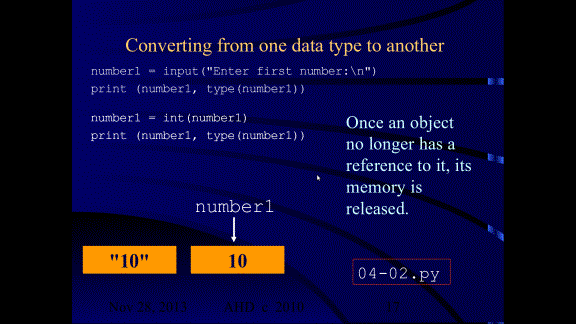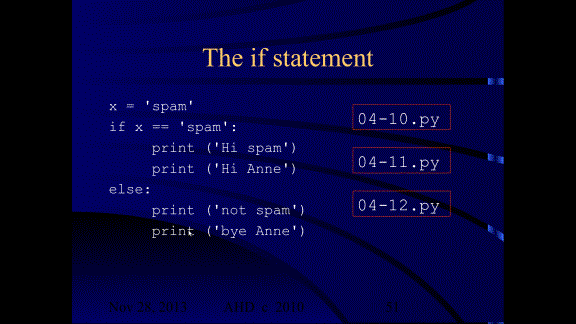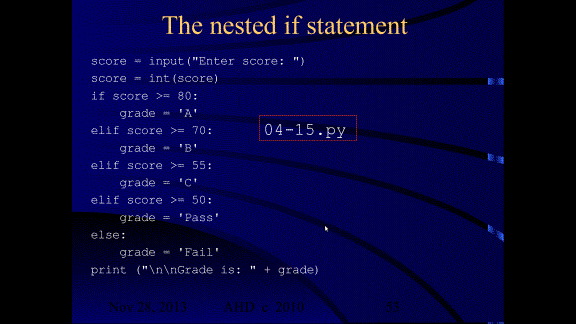```

```

# Python program statements cannot change the value of a number or string object. These objects are said to be immutable.see program:

04-01.py

For more notes on Python 3 input, see:see program:

04-02.py

### Displaying an object's memory location

A representation of the memory location of an object can be obtained by using the id function.

number1 = input("Enter first number:\n")

print (number1, type(number1), id(number1))

number1 = int(number1)

print (number1, type(number1) ), id(number1))

see program:

04-03.py

# Numeric Expressions (int)

## 3**2     # 3 to the power of 2

```The % (modulus or modulo) operator yields the remainder from the division of the first argument by the second. The arguments may be floating point numbers, e.g., 3.14  %  0.7 equals 0.34 (since 3.14 equals 4 * 0.7 + 0.34.), ```

```or integer numbers, e.g., 5 % 2 equals 1 (since 5 equals 2 * 2 + 1.).```

`` ``

```Click here for info on when you might want to use the modulus operator.```

see program:

04-04.py

# Numeric Expressions (float)

## 3.0**2.0     # 3.0 to the power of 2.0

see program:

04-05.py

```The % (modulus or modulo) operator yields the remainder from the division of the first argument by the second. The arguments may be floating point numbers, e.g., 3.14  %  0.7 equals 0.34 (since 3.14 equals 4 * 0.7 + 0.34.), ```

```or integer numbers, e.g., 5 % 2 equals 1 (since 5 equals 2 * 2 + 1.).```

`` ``

```Click here for info on when you might want to use the modulus operator.```

# Mixed Numeric Expressions

## 3**2.0     # 3 to the power of 2

```The % (modulus or modulo) operator yields the remainder from the division of the first argument by the second. The arguments may be floating point numbers, e.g., 3.14  %  0.7 equals 0.34 (since 3.14 equals 4 * 0.7 + 0.34.), ```

```or integer numbers, e.g., 5 % 2 equals 1 (since 5 equals 2 * 2 + 1.).```

`` ``

```Click here for info on when you might want to use the modulus operator.```

see program:

04-06.py

# Relational operators relate two operands

## 4 != 4

These are Boolean expressions.

The result of these expressions is either true (1) or false (0).

see program:

04-07.py

see program:

04-08.py

see program:

04-09.py

# Truth Tables of Boolean Operators

### Precedence Rules

Highest precedence

## ( )      (anything in brackets is done first)

**     (exponentiation)

## logical or

Lowest precedence

What the above means is explained in the examples below:

Example 1

````x = 17 / 2 * 3 + 2`

```
` `
``print (x)``
`` ``
`In the expression to evaluate the value of x, the operators / and * share equal precedence `
`that is higher than that of the + operator.  `
`Hence the division and multiply are done before the addition, `
`resulting in an output of  27.5, as explained here...`
` `
`17 / 2  is  8.5`
`8.5  *  3  is  25.5`
`25.5  +  2  is  27.5`
` `
` `
` `
`Example 2`
` `
````x = 2 + 17 / 2 * 3`

```
` `
``print (x)``
`` ``
`In the expression to evaluate the value of x, the operators / and * share equal precedence`
`that is higher than that of the + operator.  `
`Hence the division and multiply are done before the addition, `
`resulting in an output of  27.5, as explained here...`
`` ``
`17 / 2  is  8.5`
`8.5 * 3  is  25.5`
`25.5 + 2  is  27.5`
`` ``
`` ``
`` ``
`` ``
`Example 3`

` `

```The % (modulus or modulo) operator yields the remainder from the division of the first argument by the second. The arguments may be floating point numbers, e.g., 3.14  %  0.7 equals 0.34 (since 3.14 equals 4 * 0.7 + 0.34.), ```

```or integer numbers, e.g., 5 % 2 equals 1 (since 5 equals 2 * 2 + 1.).```

`` ``
``Click here for info on when you might want to use the modulus operator.``
`` ``
``x = 19 % 4 + 15 / 2  * 3``
``print (x)``
`` ``
`In the expression to evaluate the value of x, the operators % / and * share equal precedence `
`that is higher than that of the + operator. `
`Hence the % (modulus), division and multiply are done before the addition, `
`resulting in an output of  25.5, as explained here...`
`` ``
`19  %  4   is  3`
`15 / 2  is  7.5`
`7.5  *  3   is  22.5`
`22.5  +  3  is  25.5`
`` ``
`` ``
`` ``
`` ``
`Example 4`
`` ``
``x = (15 + 6) - 10 * 4``
``print (x)``
`` ``
`In the expression to evaluate the value of x, the brackets have highest of all precedence, `
`so is evaluated before anything else, then * is done, and lastly the - subtraction, `
`resulting in an output of  -19 (minus 19), as explained here...`
`` ``
`15 + 6  is  21`
`10 * 4  is  40`
`21 - 40 is -19`
` `
` `
` `
` `
` `
`Example 5`

````x = 17 / 2 % 2 * 3**3`

```
` `
``print (x)``
`` ``
`In the expression to evaluate the value of x, the exponentiation is done first `
`(three to the power of 3 is 3 * 3 * 3 which equals 27) as it has higher precedence to the other operators (/ % and *). `
` `
`So, ...`
` `
`This is the order of evaluation:`
`Exponentiation is done first and the expression then evaluates from left to right:`
`3 to the power of 3 is 27. `

the expression is now 17 / 2 % 2 * 27

17 / 2  is  8.5

8.5 % 2 is 0.5

0.5 * 27  is 13.5

```

```
```

```

# The if statement

The if statement starts with the keyword if followed by a Boolean expression, followed by a colon (:).

## print ('not spam')

Beneath the if line, the statements to be run if the condition is true are entered after pressing the Tab key or typing a few space characters. If you use the Tab key, always use the Tab key. If you use three spaces, always use three spaces. Mixing Tabs and spaces is a syntax error, even if the program looks correct! You will get an "incorrect indent" error. You have been warned!

The if statement makes use of a Boolean expression to decide which statement(s) to execute.

```
```
```
```

The Boolean expression in this example is:

x == 'spam'

The expression has a value of true or false (1 or 0).

The Boolean expression is also known as the condition of the if statement.

If the condition is true,

the first print statement is executed and the second one is skipped.

If the condition is false,

the first print statement is skipped and the second one is executed.

It's possible to have multiple statements in the true or false sections

of an if statement...

## print ('bye Anne')

If the condition is true, the first two print statements are executed

and the second set are skipped.

# The if statement - syntax

The if statement starts with the keyword if followed by a Boolean expression, followed by a colon (:).

## print ('not spam')

Beneath the if line, the statements to be run if the condition is true are entered after pressing the Tab key or typing a few space characters.

The statements to be run must be indented to the same level.

It's recommended to press the Tab key before typing the statements.

If you use the Tab key, always use the Tab key. If you use three spaces, always use three spaces. Mixing Tabs and spaces is a syntax error, even if the program looks correct! You will get an "incorrect indent" error. You have been warned!

## print ('not spam')The else part of an if statement is optional, but if included, must be followed by a colon (:), and then the indented statement(s) to be executed if the condition is false.

see programs:

04-10.py

04-11.py

04-12.py

# The nested if statement

Nested if/else statements test for multiple cases

by placing if/else selection structures inside other

if/else selection structuressee programs:

04-13.py

04-14.py

# The nested if/elif/else statement

Nested if/else statements can be written using an alternateif/elif/else construct.

Program 04-14.py is exactly equivalent to 04-15.py.

see program:

04-15.py

04-17.py

```
```
```
```

# 4.6 De Morgan's Laws (Reference only)

(You will NOT be tested on De Morgan's Laws on this course)

## Wiki - De Morgan's Laws

1. A not and is equivalent to an or with two negated inputs.

2. A not or is equivalent to an and with two negated inputs.

see program:

04-16.py

04-01.py

04-02.py

04-03.py

04-04.py

04-05.py

04-06.py

04-07.py

04-08.py

04-09.py

04-10.py

04-11.py

04-12.py

04-13.py

04-14.py

04-15.py

04-16.py

04-17.py